• 公式复杂，但详尽吃透可以学习到SVGD、EBM等概念与算法
• 文章充实，可以继续阅读后续算法SAC
• 拓展在强化学习与熵进行结合方面的知识

# 简介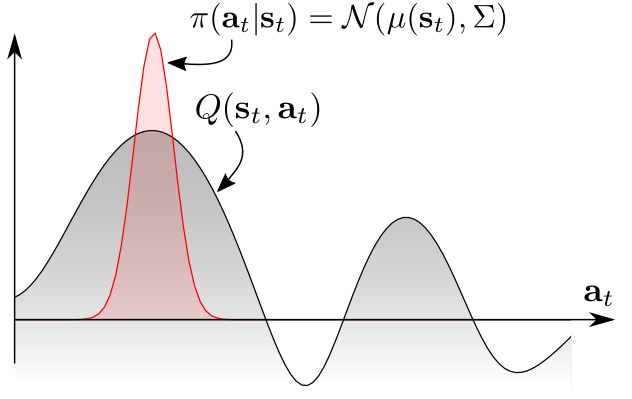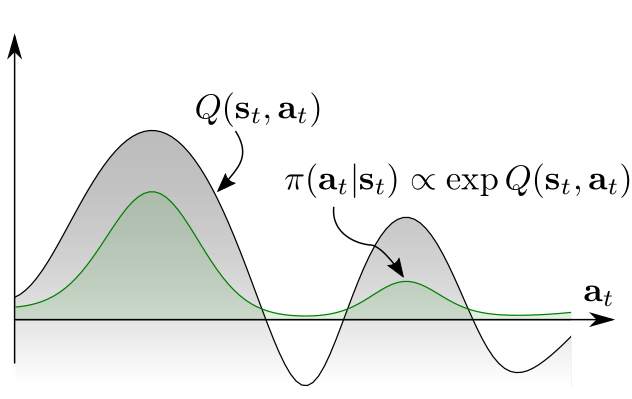As discussed in prior work, a stochastic policy emerges as the optimal answer when we consider the connection between optimal control and probabilistic inference.

• 如果可以全面地学习给定任务中的目标策略，那么结果策略可以作为很好的初始化策略，微调后以学习更高级的策略
• 这种随机的探索机制，可以更好地寻求多峰任务中的最佳决策模型
• 更好的鲁棒性，环境有干扰或者噪音时，有多种完成目标的行动可以选择，可以从干扰中“脱身”

## 算法效果

The applications of training such stochastic policies include improved exploration in the case of multimodal objectives and compositionality via pretraining general-purpose stochastic policies that can then be efficiently finetuned into task-specific behaviors.

# 文中精要

## 标准强化学习的最优策略

• std下标代表标准的意思：standard，星号$\ast$代表最优

• $\rho_{\pi}$代表策略$\pi\left(\mathbf{a}_{t} | \mathbf{s}_{t}\right)$下的迹分布，$\rho_{\pi}\left(\mathbf{s}_{t}, \mathbf{a}_{t}\right)$代表状态-行动对的边缘分布。

We will also use $\rho_{\pi}\left(\mathbf{s}_{t}\right)$ and $\rho_{\pi}\left(\mathbf{s}_{t}, \mathbf{a}_{t}\right)$ to denote the state and state-action marginals of the trajectory distribution induced by a policy $\pi\left(\mathbf{a}_{t} | \mathbf{s}_{t}\right)$.

## 最大熵强化学习的最优策略

• MaxEnt下标代表最大熵的意思：Maximum entropy
• 式中的系数$\alpha$可以用来调节奖励项与熵值项的重要性比率。一般将$\alpha$表示为奖励范围（reward scale）的倒数，但在实际中通常将其作为超参数手动调节。SAC算法中有介绍在训练过程中自动调节该系数的方法。
• 本文中的SQL算法也是为了优化该目标函数

## 最大熵目标的优点

• 在多峰（即一个状态下有多个最优动作选择）问题中提升探索能力
• 可以用于迁移学习，因为其“预训练”模型更好地适应之后的任务

soft Q函数定义如下：

soft V函数定义如下：

## 能量模型与策略

• $\mathcal{E}$是字母E的花体形式，代表能量函数，其可以被深度神经网络表示，如果使用通用值函数近似来表示能量函数，那么可以表示任意策略$\pi\left(\boldsymbol{a}_{t} | \mathbf{s}_{t}\right)$

• where $\mathcal{E}$ is an energy function that could be represented, for example, by a deep neural network. If we use a universal function approximator for $\mathcal{E}$, we can represent any distribution $\pi\left(\boldsymbol{a}_{t} | \mathbf{s}_{t}\right)$.

• 文中将该能量函数设置为

其实也很容易就能理解，将负号抵消掉之后，Q值大的动作能量高嘛，指数分布又能更好的放大较大的值，使Q值大的动作更为突出，这样完全可以作为选择动作的策略

BlueFisher's Blog

## 使用SQL优化目标函数

### Soft Q-Iteration

1. 连续空间无法求期望，或者计算不准确。解决方案是重要性采样，使用采样多次后计算来代替积分，在初期进行随机均匀采样，后期根据policy来采样。
2. 迭代过程需要不断选择动作，问题是式（8）的分布形式无法进行采样。解决方案是使用SVGD算法拟合后验分布，并输出采样的动作。

### 近似采样与SVGD

1. MCMC采样，即马尔科夫链蒙特卡洛采样
2. 学习一个采样网络去近似采样出符合目标分布的样本

Amortized SVGD论文：https://arxiv.org/abs/1707.06626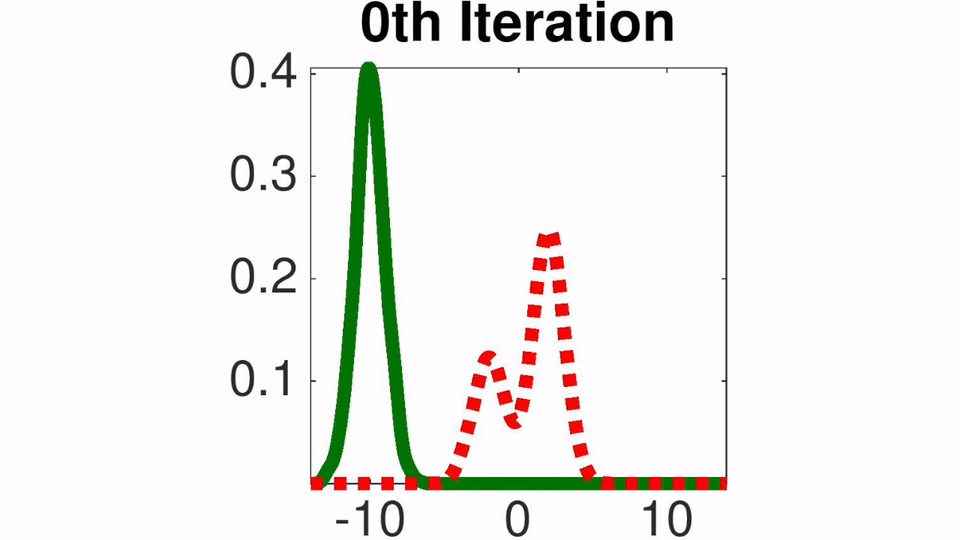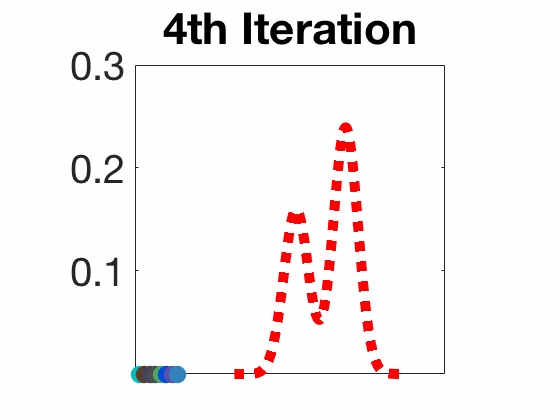Amortized SVGD有一些有趣的性质：

• 可以训练随机采样网络非常快地采样
• 可以准确收敛至EBM能量模型的后验估计分布
• 文中结合了Amortized SVGD后，算法形式很像A-C模式

SVGD算法的更新形式是这样的，

• $\epsilon$代表学习率
• $k\left(x_{j}, x_{i}\right)$代表正定核，如径向基（RBF，Radial Basis Function）函数$k\left(x, x^{\prime}\right)=\exp \left(-\frac{1}{h}\left|x-x^{\prime}\right|_{2}^{2}\right)$，它可以被认为是变量之间的相似性度量
• 包含对数项$\log p\left(x_{j}\right)$的梯度驱使采样器朝着$p(x)$分布中高概率区域进行采样
• 第二项核函数梯度驱使样本点之间产生间隙，相当于用一个排斥力使样本点尽可能分散开
• 对数项梯度不依赖分布$p(x)$的归一化常数，使SVGD易于应用于图模型、贝叶斯推理和深层生成模型中出现的难以处理的分布。

• $\phi$表示采样网络中的参数
• 将产生动作的函数简写成$\mathbf{a}_{t}^{(i)}=f^{\phi}\left(\xi^{(i)} ; \mathbf{s}_{t}\right)$，也就是说神经网络的输入分为两部分，一部分是状态$s$，一部分是噪声扰乱“perturb”$\xi$，一般从标准正态分布中采样，而且最好使噪声的维度与动作的维度一致

• 严格来说，$\Delta f^{\phi}$是希尔伯特空间的最优梯度方向，并不是Actor目标函数$J_{\pi}$的梯度

• To be precise, $\Delta f^{\phi}$ is the optimal direction in the reproducing kernel Hilbert space of $\kappa$, and is thus not strictly speaking the gradient of $J_{\pi}\left(\phi ; \mathbf{s}_{t}\right)$

## 伪代码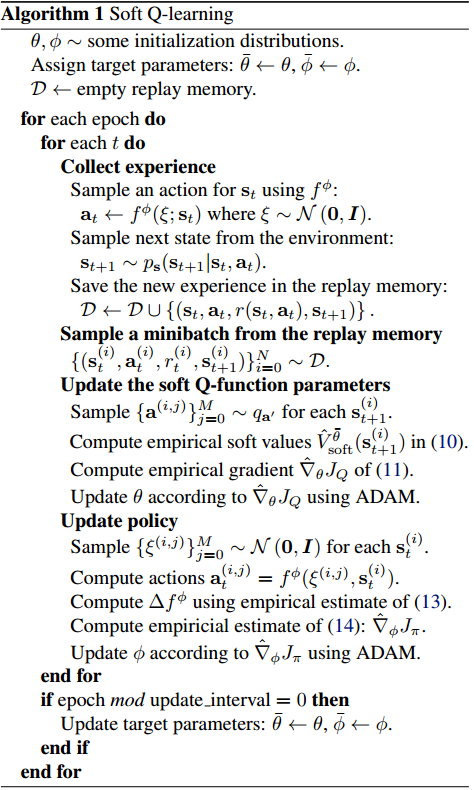• 伪代码中定义了Actor的target网络，参数为$\overline{\theta}$。但是伪代码中并没有显示出其在何处使用，我猜测该网络代表采样分布$q_{\mathbf{a}^{\prime}}$
• $q_{\mathbf{a}^{\prime}}$在训练初期使用均匀分布
• $q_{\mathbf{a}^{\prime}}$在一段时间之后使用Actor真实分布，我猜测这里使用的就是Actor目标网络
• 噪音$\xi$从多维标准正态分布中采样，维度最好与动作空间维度一致
• $\left\{\mathbf{a}^{(i, j)}\right\}_{j=0}^{M} \sim q_{\mathbf{a}^{\prime}}$其中的M用于设置采样多少个样本，以使用公式（11）计算V值，使用的网络为Q目标网络
• 在更新Q网络时，使用了从经验池采样到的真实执行过的动作
• $\left\{\xi^{(i, j)}\right\}_{j=0}^{M} \sim \mathcal{N}(\mathbf{0}, \boldsymbol{I})$中其实少写了一个参数$K$，但实际上$K=M$，在这一步中需要采样两组噪音，当然也可以采样一组，使用两次。
• 在更新Actor网络时，没有使用经验池中采样到的动作，而模拟采样了两组动作，即根据两组噪音生成的动作，用它们来计算梯度并更新。
• Q网络的输入为状态与动作的连接，$(s||a)$，输出为Q值
• Actor网络的输入为状态与噪音的连接，$(s||\xi)$，输出为动作$a$
• 伪代码中的式(10)、(11)、(13)、(14)分别代表本文中的式(11)、(12)、(16)、(17)

# 实验

## 实验设置

• 比较算法：DDPG vs SQL
• Actor学习率为0.0001，Q网络学习率为0.001
• 经验池大小为100W
• 经验池填充1W条经验后开始训练
• batch_size=64
• Actor和Q网络都是2层隐藏层，每层200个隐藏节点，激活函数为ReLU
• DDPG和SQL都使用了Ornstein-Uhlenbeck随机过程产生噪音来增加探索，它是一种序贯相关的随机过程，$\theta=0.15 \ , \ \sigma=0.3$

• OU随机过程可以在序贯模型中添加与时间相关的随机噪音，而且噪音也满足强马尔可夫性

• 形式为$d x_{t}=\theta\left(\mu-x_{t}\right) d t+\sigma d W_{t}$，是一个具有均值恢复属性的随机过程

• $\theta$表示变量$x$以多大幅度、多块恢复到平均值，$\mu$代表平均值，$\sigma$代表波动程度，$d W_{t}$代表维纳过程，一般通过高斯分布实现

• OU随机过程产生的噪音只与上一次产生的噪音相关，它可以用于增加探索，也能够柔顺控制。比如在相邻的两个决策动作，一个为10，一个为-10，反复如此，智能体会产生震荡。在此使用OU过程可以使智能体在一个方向保持一定时间，不会瞬间过大地改变智能体的状态，相当于增加了时滞性。

• 代码

# 个人感想

-------------本文结束感谢您的阅读-------------

0%